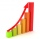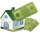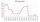# Expression of a variable from the formula + interest, deposits and loan math - math problems

#### Number of problems found: 6

• Semiannually compound interestIf you deposit \$5000 into an account paying 8.25% annual interest compounded semiannually, how long until there is \$9350 in the account?
• Slow saving in banksHow long will it take to save € 9,000 by depositing € 200 at the beginning of each year at 2% interest?
• Compound interest 43600 dollars is placed in an account with an annual interest rate of 9%. How much will be in the account after 25 years, to the nearest cent?
• Mortage hypo loanThe Jonáš family decided to buy an older apartment, which cost EUR 30,000. They found EUR 17,000 and took the loan with the bank for the remaining amount. What interest did they receive if they repaid this amount for 15 years at EUR 120 per month?
• Interst on savingsThe bank offers 1.6% interest. How many euros have to insert at the beginning if we received € 15 on the interest?
• The depositDeposit has been placed for one year at an annual rate of 4.5%. After the added interst amount rose to € 2,612.5. Determine initial deposit.

We apologize, but in this category are not a lot of examples.
Do you have an exciting math question or word problem that you can't solve? Ask a question or post a math problem, and we can try to solve it.

We will send a solution to your e-mail address. Solved examples are also published here. Please enter the e-mail correctly and check whether you don't have a full mailbox.

Expression of a variable from the formula - math problems. Interest, deposits and loan math - math problems.